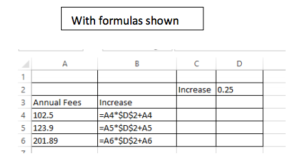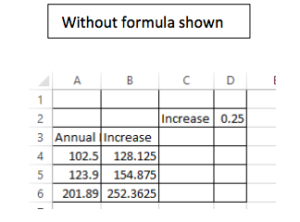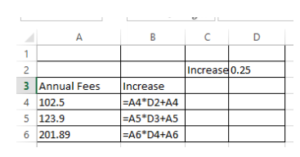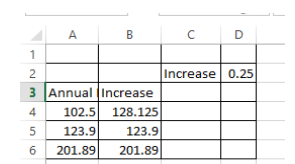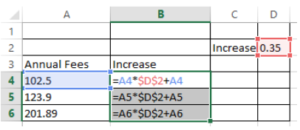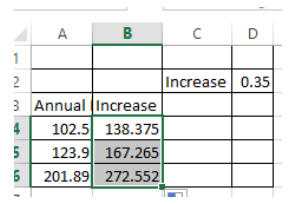## Relative and Absolute Referencing

### Student Learning Outcome:

The student will understand the difference between Relative and Absolute Referencing and apply this information in an assignment.

Relative Referencing is what you normally do in a spreadsheet. If you add a column of numbers, (for example), and then pull the formula to the right, you are using a relative reference.

See illustration below: By using A1:A4, the formula adds column A: (A1+A2+A3+A4). Pulling the formula to the right into column B automatically changes the column reference letters to “B” without you having to do anything.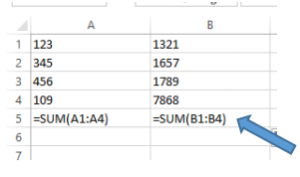To “pull” a formula, or move it into the next cell, move your insertion point (the arrow) – to the bottom right corner of the cell with the formula. There should be a small square box in that corner. (Notice where the arrow is pointing the illustration.) When the arrow turns into a black plus sign, left click and hold down the left mouse. Then drag that box to the right. When you release the mouse, the formula has been placed in the next column and has automatically changed the letters.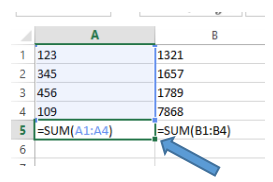Relative Reference means that the cells are relative – have a relationship with other cells. The spreadsheet program has created this relationship.

Absolute Referencing interrupts the relative referencing so that only chosen cell references can be used.
Using the dollar sign (\$) makes a reference absolute.

Examples of absolute references.

1.)=A1 = relative reference

2.)=\$A1 = Column is absolute, row is relative

3.)=A\$1 = Row is absolute, column is relative

4.)=\$A\$1 = Everything is absolute

TRY THIS to understand how it works:

1. Open a blank spreadsheet and type in the annual Fees as noted in cells A4, A5, and A6.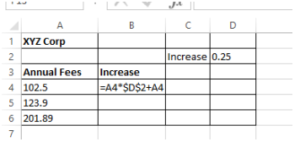2. In cell B4 type the formula as you see it here. (Note: the dollar signs – which make this reference absolute – are created by first clicking on cell D2 then pressing the F4 key which is located at the very, very top of the keyboard. There are other keys in this row such as F1 – which is the help key -, F2, F3, F5, etc. When F4 is pressed, dollar signs should appear before the column reference and before the row reference – in this case \$D\$2. (If the F4 key doesn’t appear to work, make sure that the referenced cell (D2) is highlighted. Or you can type the dollar signs by hand.)

3. Press enter. The amount of 128.125 appears.

4. Additional Note: Why put in the cell reference C4 two times? This is because you are taking the A4 reference and multiplying it by 25%. Then you adding the original amount back in to get the whole increase amount. You are basically taking 1.25% of the Annual fee amount. (The math is like this: (A4) \$102.50* (\$D\$2).25% (.25) = 25.625. This is the amount of the increase. Then you are adding back in the number you started with – the original annual fee of 102.50 to determine the total amount of the increase (25.625+102.50 = 128.125.

5. Pull the formula in B4 down to include B6. Look at the formula carefully. Notice that the Annual fee reference changed because it is relative (or references the next cell). However, the absolute reference (D2) did not change. If the dollar signs were removed, the formula in B5 would be =B5*D3+B5 because it would be relative, meaning it would go to the next cell (D3). The answer would not contain any increase because D3 cell reference is blank.